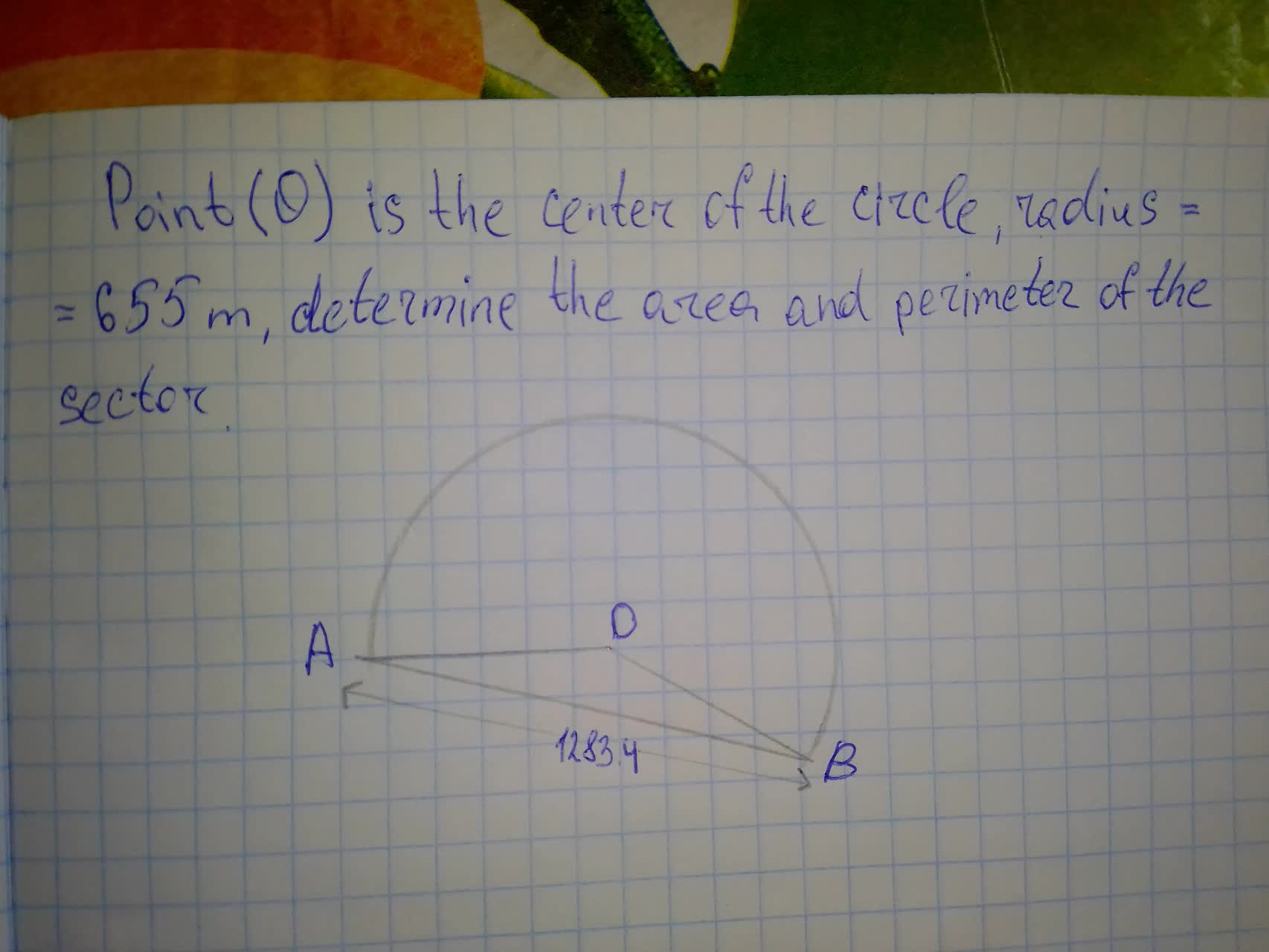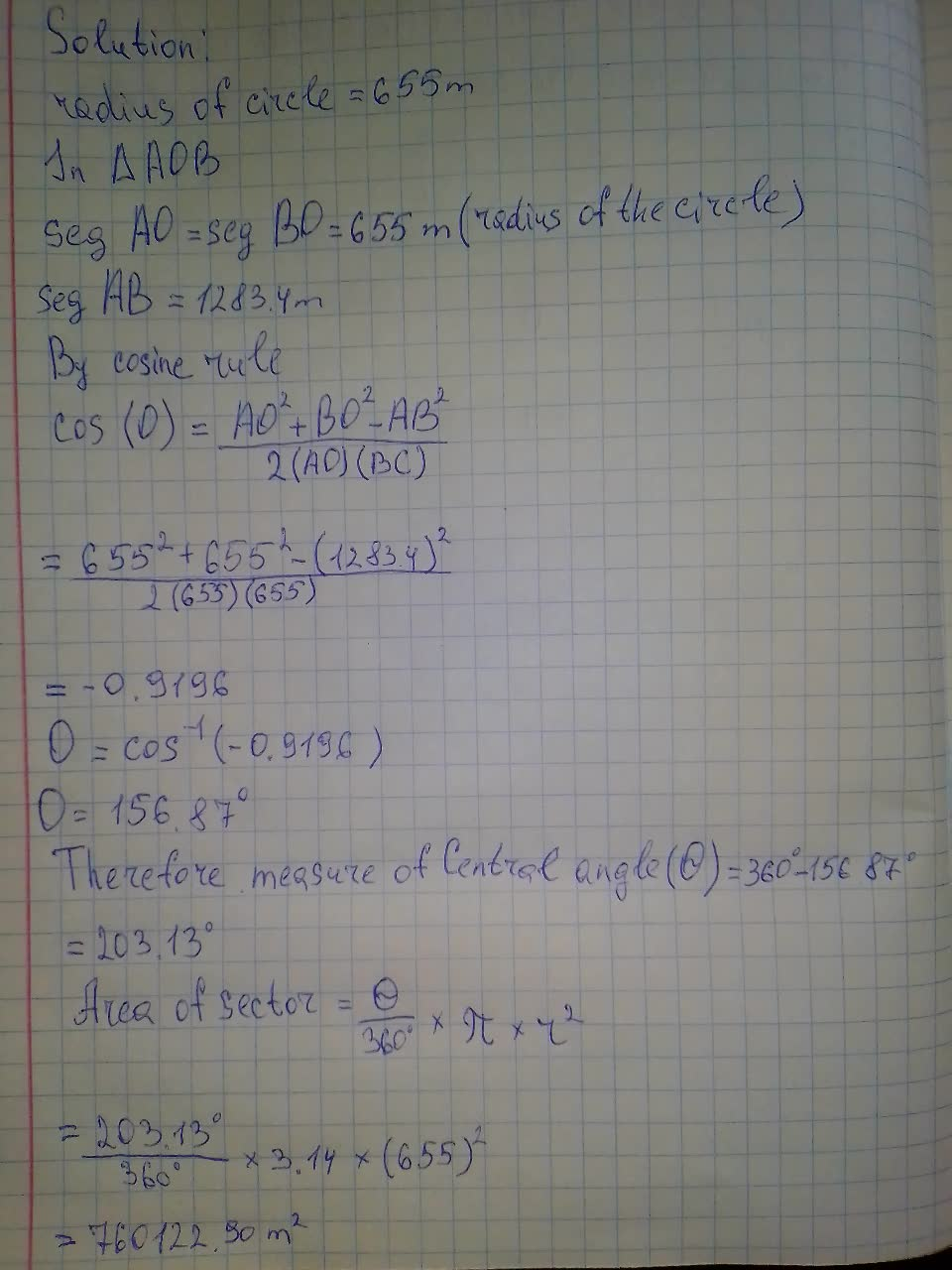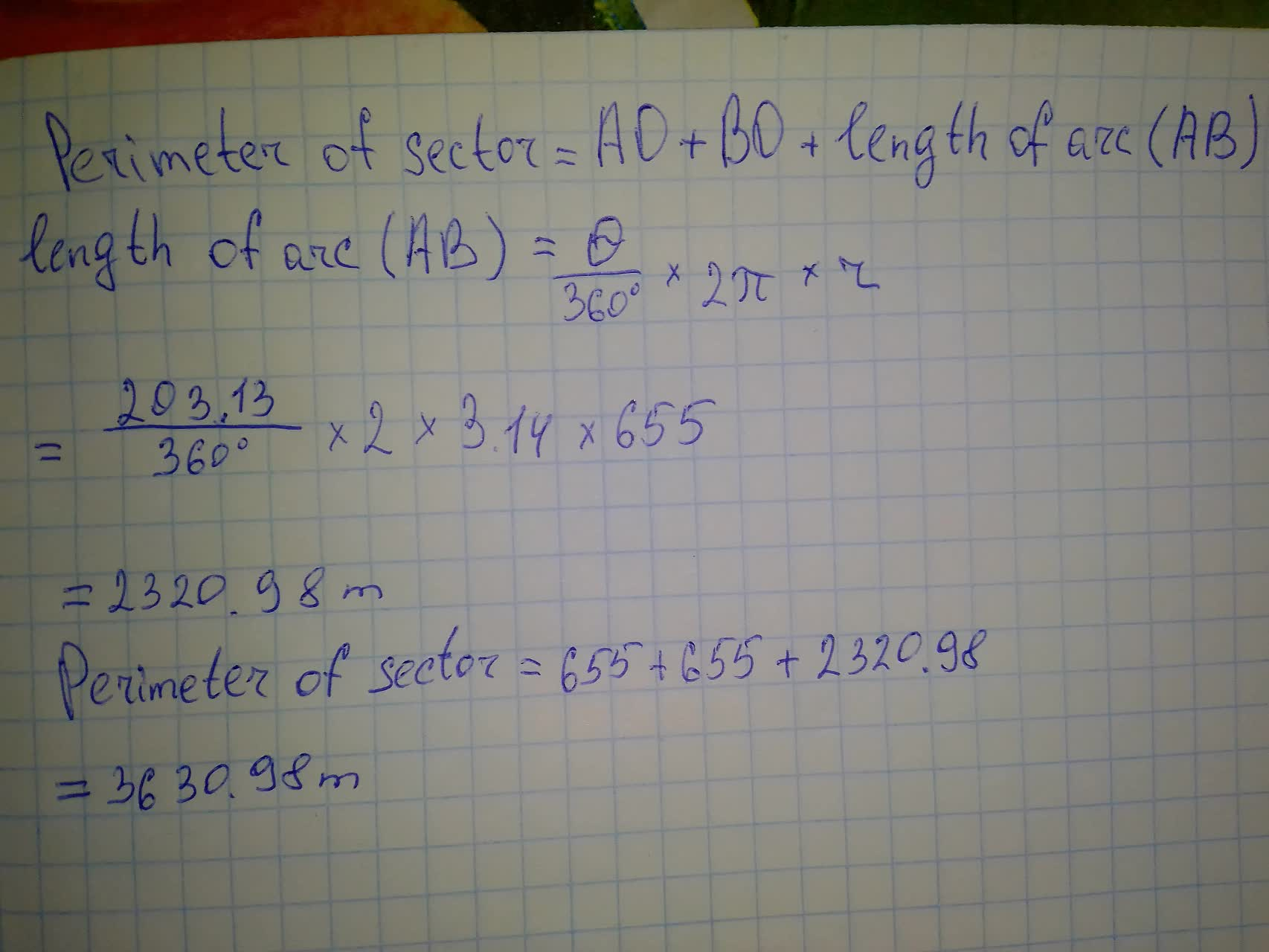# Point (O) is the center of the circle, radius = 655 m, determine the area and perimeter of the sector.alesterp 2021-08-11 Answered
Point (O) is the center of the circle, radius = 655 m, determine the area and perimeter of the sector.You can still ask an expert for help

• Questions are typically answered in as fast as 30 minutes

Solve your problem for the price of one coffee

• Math expert for every subject
• Pay only if we can solve itDora

Solution of this problem